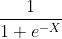Join thousands of AI enthusiasts and experts at the Learn AI Community.

## Publication# Logistic Regression Explained Simply

Last Updated on December 21, 2020 by Editorial Team

#### Machine Learning, Statistics

Logistic regression is a technique borrowed by machine learning from the field of statistics. It is the go-to method for binary classification problems (problems with two class values).

### Logistic Function

Logistic regression is named for the function used at the core of the method, the logistic function.

The logistic function, also called the sigmoid function was developed by statisticians to describe properties of population growth in ecology, rising quickly and maxing out at the carrying capacity of the environment. It is an S-shaped curve that can take any real-valued number and map it into a value between 0 and 1, but never exactly at those limits.

where e is the base of the natural logarithms and X is the actual numerical value that you want to transform. Below is a plot of the numbers between -5 and 5 transformed into the range 0 and 1 using the logistic function.

### Representation Used for Logistic Regression

Logistic regression uses an equation as the representation, very much like linear regression.

Input values (X) are combined linearly using weights or coefficient values (referred to as the Greek capital letter Beta) to predict an output value (y). A key difference from linear regression is that the output value being modeled is a binary values (0 or 1) rather than a numeric value.

Below is an example logistic regression equation:

where y is the predicted output, b0 is the bias or intercept term and b1 is the coefficient for the single input value (X). Each column in your input data has an associated b coefficient (a constant real value) that must be learned from your training data.

The actual representation of the model that you would store in memory or in a file are the coefficients in the equation (the beta value or b’s).

### Logistic Regression Predicts Probabilities (Technical Interlude)

Logistic regression models the probability of the default class (e.g. the first class).

For example, if we are modeling people’s sex as male or female from their height, then the first-class could be male and the logistic regression model could be written as the probability of male given a person’s height, or more formally:

Written another way, we are modeling the probability that an input (X) belongs to the default class (Y=1), we can write this formally as:

Note that the probability prediction must be transformed into binary values (0 or 1) in order to actually make a probability prediction. Logistic regression is a linear method, but the predictions are transformed using the logistic function. The impact of this is that we can no longer understand the predictions as a linear combination of the inputs.

### Prepare Data for Logistic Regression

The assumptions made by logistic regression about the distribution and relationships in your data are much the same as the assumptions made in linear regression. Much study has gone into defining these assumptions and precise probabilistic and statistical language is used.

Ultimately in predictive modeling machine learning projects, you are laser-focused on making accurate predictions rather than interpreting the results. As such, you can break some assumptions as long as the model is robust and performs well.

• Binary Output Variable: This might be obvious but logistic regression is intended for binary (two-class) classification problems. It will predict the probability of an instance belonging to the default class, which can be snapped into a 0 or 1 classification.
• Remove Noise: Logistic regression assumes no error in the output variable (y), consider removing outliers and possibly misclassified instances from your training data.
• Gaussian Distribution: Logistic regression is a linear algorithm (with a non-linear transform on output). It does assume a linear relationship between the input variables with the output. Data transform of your input variables that better expose this linear relationship can result in a more accurate model.
• Remove Correlated Inputs: Like linear regression, the model can overfit if you have multiple highly-correlated inputs. Consider calculating the pairwise correlations between all inputs and removing highly correlated inputs.
• Fail to Converge: It is possible for the expected likelihood estimation process that learns the coefficients to fail to converge. This can happen if there are many highly correlated inputs in your data or the data is very sparse (e.g. lots of zeros in your input data).

There is a lot of material available on logistic regression. It is a favorite in may disciplines such as life sciences and economics.

### Logistic Regression Resources

Check out some of the books below for more details on the logistic regression algorithm.

### Logistic Regression in Machine Learning

For a machine learning focus (e.g. on making accurate predictions only), take a look at the coverage of logistic regression in some of the popular machine learning texts below:Logistic Regression Explained Simply was originally published in Towards AI on Medium, where people are continuing the conversation by highlighting and responding to this story.

Published via Towards AI